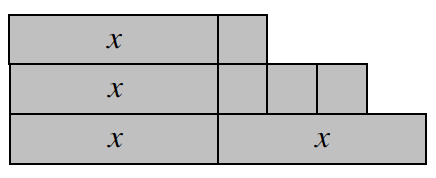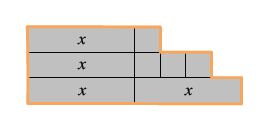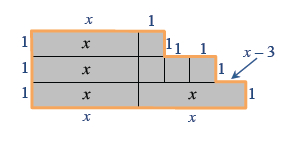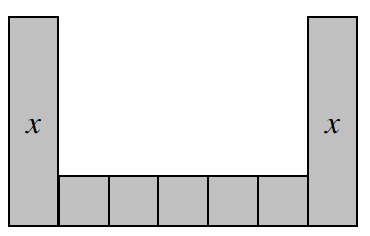### Home > CCAA > Chapter 6 Unit 4 > Lesson CC2: 6.1.3 > Problem6-34

6-34.

Find the perimeter and area of each algebra tile shape below. Be sure to combine like terms.

1.Remember, the perimeter is the sum of all the side lengths around the shape. Algebra Tile Diagram: A line is added that is drawn around the perimeter of the figure.

Labeling all the side lengths may prove to be helpful for you.  Labels added to the sides of the tiles, starting at the top left, and going clockwise: x, 1, 1, 1, 1, 1, x minus 3, 1, x, x, 1, 1, 1

Add up all these values to find the perimeter.

The area is the space within the orange lines.

Add up the values of the algebra tiles to find the area.

$P=4x+6$
$A=4x+4$

1.See part (a).